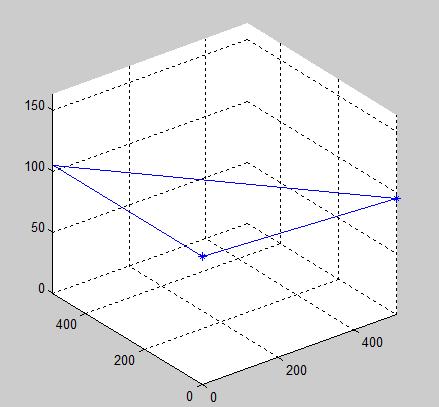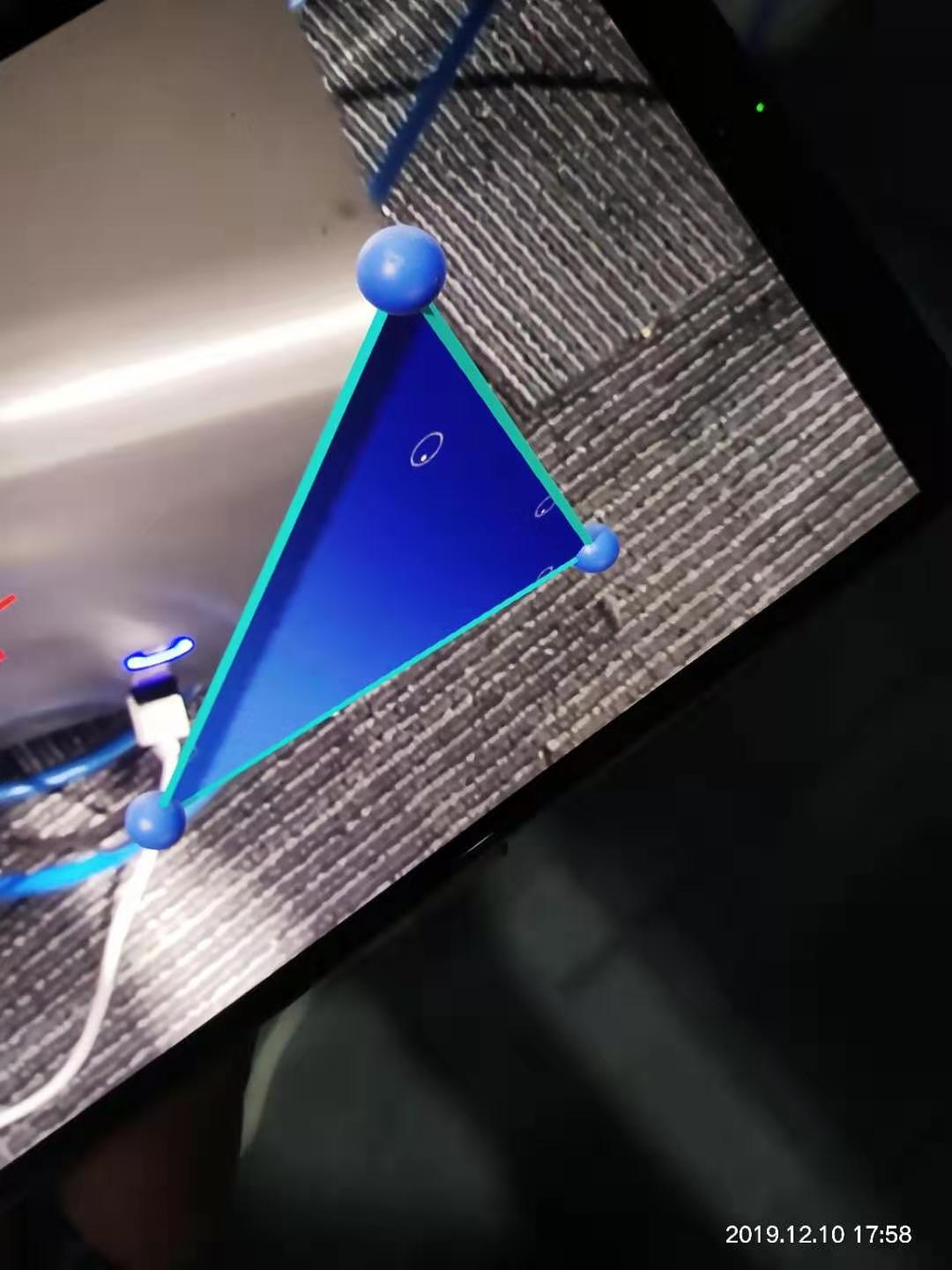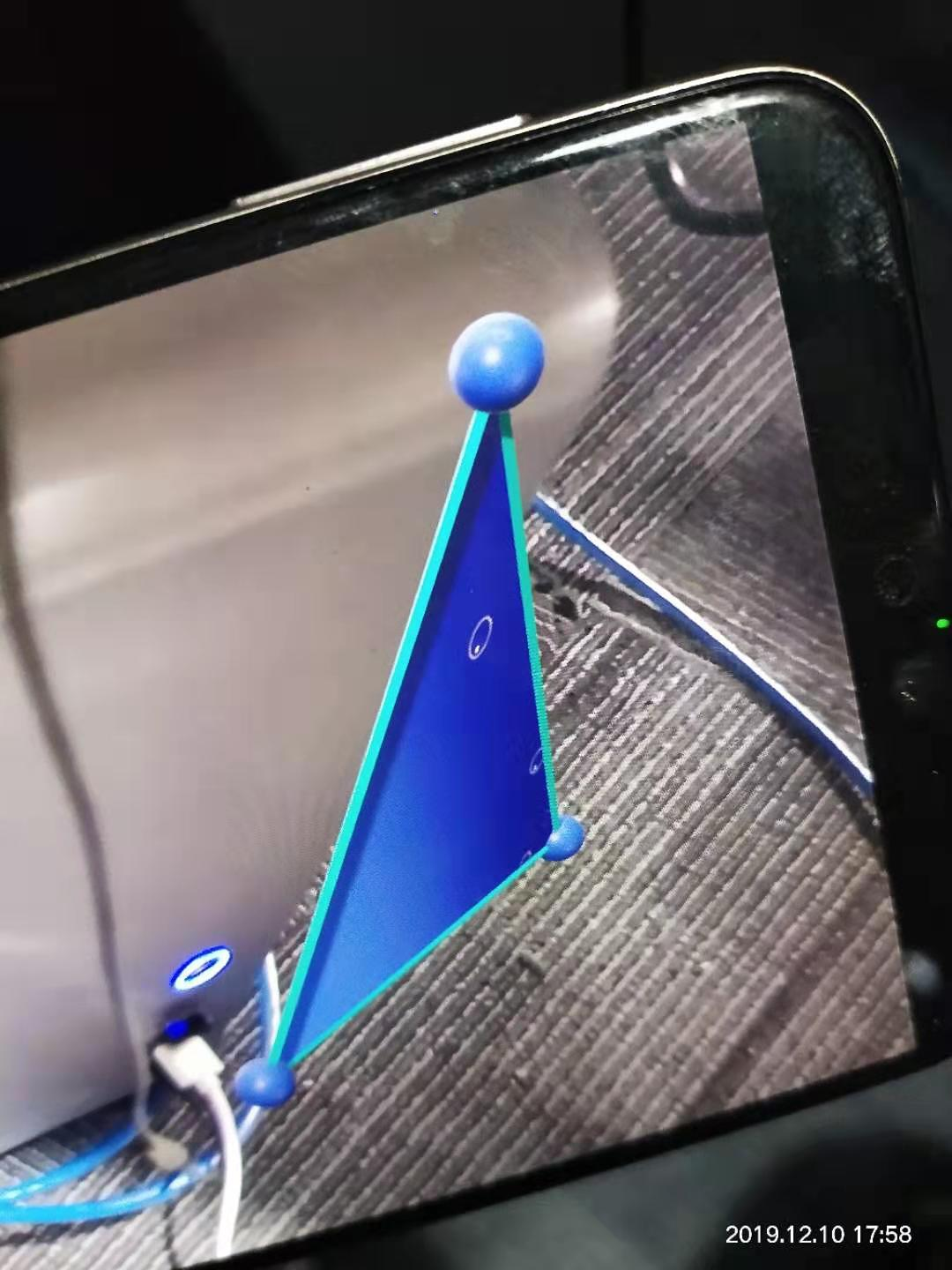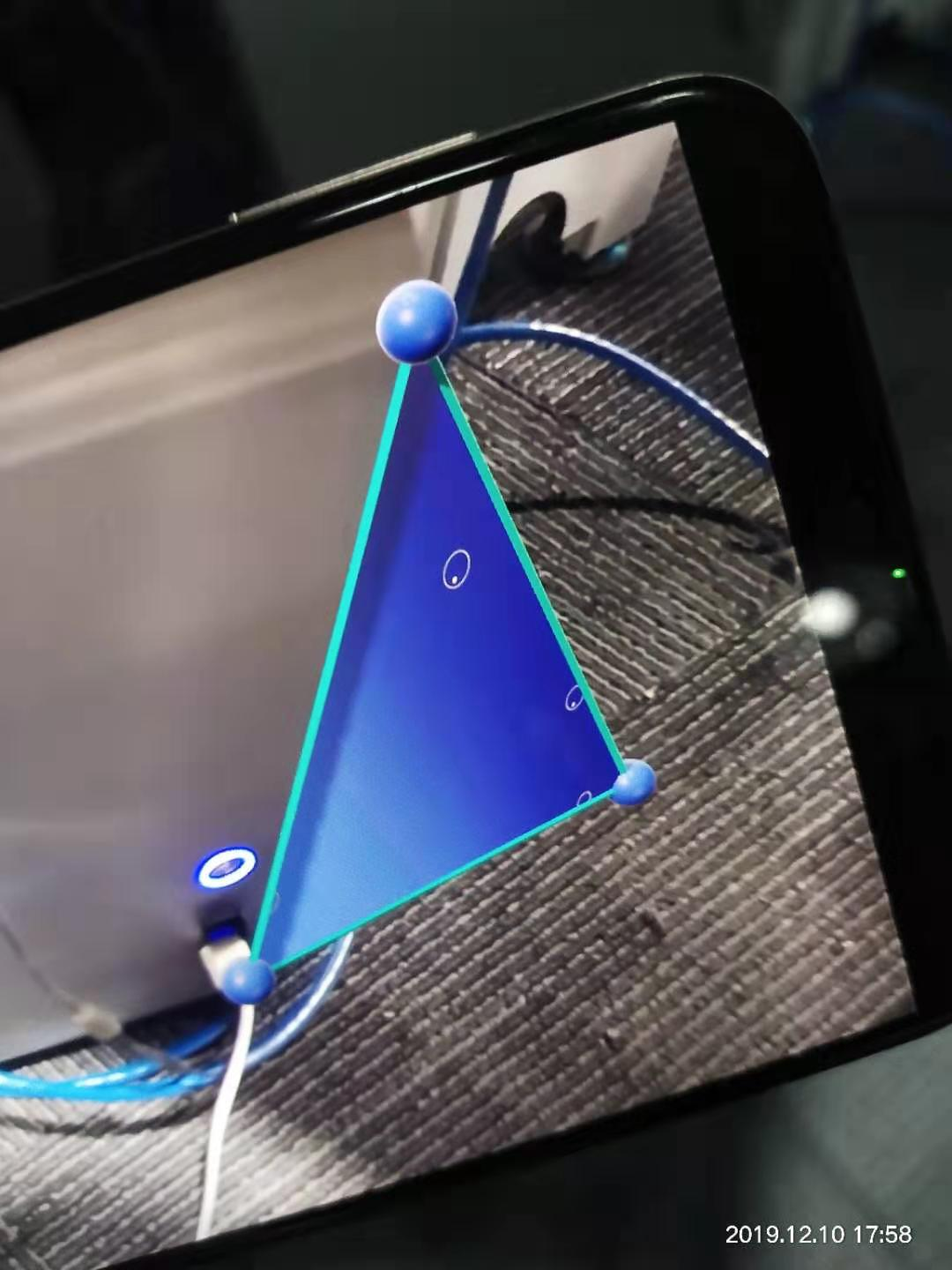• 已知三点p1,p2,p3,求其平面方程，p1=[x1,y1,z1];p2=[x2,y2,z2];p3=[x3,y3,z3];那么求解下面矩阵q行列式就是了：q=[ones(4,1),[x,y,z];p1;p2;p3];detb=det(q);最后令q=0; 这里的求解方法是四阶矩阵的行列式求解，可以...
1.基本原理

已知三点p1,p2,p3,求其平面方程，p1=[x1,y1,z1];p2=[x2,y2,z2];p3=[x3,y3,z3];那么求解下面矩阵q行列式就是了：q=[ones(4,1),[x,y,z];p1;p2;p3];detb=det(q);最后令q=0;
这里的求解方法是四阶矩阵的行列式求解，可以从三阶矩阵的行列式推导出来，那就是常规的求解方法：方程组写成[p1;p2;p3]=D,其中D=[d,d,d]'表示平面方程的常数项，先求q=[p1;p2;p3]的行列式，再分别求D与三个坐标两个分量合成的三阶矩阵的行列式，设为q1,q2,q3；那么所求的平面方程的四个参数就是A=q1/q;B=q2/q;c=q3/q;D=d;同时乘以q/d所得结果本质上就是上面四阶矩阵行列式解。
2.Matlab代码
<span style="font-size:14px;">function [a,b,c,d] = TriPts2Plane( A,B,C )
%不共线三点确定一个平面
%具体指，2DUS提供的数据点确定3DCT中的一个参考平面
% A,B,C 不共线的三个点
%a,b,c,d 确定平面方程的参数
%沈春旭/沈子恒 2016.4.8
%-------------------------------Step1:确定平面方程-------------------------%
syms x y z
%D的行列式等于零就是平面方程。
if (det([A;B;C]) == 0)
msgbox('特征点线性相关，不足以构成平面');
return;
else
D=[ones(4,1),[[x,y,z];A;B;C]];
detd = det(D);
str = char(detd);
%---------------------------------Step2: 提取系数--------------------------%
amark = find(str=='x');
bmark = find(str=='y');
cmark = find(str=='z');
%-------------------------------------------
if(size(amark,2))
a = coeffs(detd,x);   a = double(a(2));%系数按照升幂顺序排列
else
a = 0;
end
%-------------------------------------------
if(size(bmark,2))
b = coeffs(detd,y);   b = double(b(2));
else
b = 0;
end
%-------------------------------------------
if(size(cmark,2))
c = coeffs(detd,z);   c = double(c(2));
else
c = 0;
end
%-------------------------------------------
[~,num] = size(str);
if(num>max([amark,bmark,cmark]))
d = coeffs(detd);     d = double(d(1));
else
d = 0;
end
%-------------------------------------------
end
end</span>


测试代码：
clc; clear all; close all;
A=[0,0,105];%A,B,C的坐标由自己定义。
B=[0,512,105];
C=[512,0,95];
[a,b,c,d]=TriPts2Plane(A,B,C);
plane = [A;B;C;A];
plot3(plane(:,1),plane(:,2),plane(:,3),'*');
hold on
%ezmesh(z)plot3(plane(:,1),plane(:,2),plane(:,3),'b-');
axis([0 512 0 512 0 163])
grid on;
测试结果：展开全文• ## 平面三点计算夹角

万次阅读 2017-02-13 21:09:14
平面给定三点，计算夹角的种方法。
纯数学问题，今天正好有人询问到，搜索复习几何知识，留此记录。
平面给定三点，计算夹角有三种方法：
1 使用向量计算
关于向量a和b，向量夹角余弦公式   a*b = |a||b|cosTheta所以    夹角 = acos(a*b) / (|a|*|b|)

两向量的数量积 = a.x*b.x + a.y*b.y
向量的模 = sqrt(x*x+y*y)
在二维下，计算量是最小的一种方法了(节约了sqrt计算)，只需要一次acos
例，三个点A(1,1), B(2,1), C(2,2)
AB=(B.x-A.x, B.y-A.y)=(1,0)
AC=(C.x-A.x, C.y-A.y)=(1,1)
cosA = (AB*AC)/(|AB|*|AC|)=1/√2=√2/2, 则夹角BAC=45度
2 余弦定理
cosA = (AB*AB + AC*AC - BC*BC ) / 2*AB*AC

例，三个点A(1,1), B(2,1), C(2,2)
cosA = (1+2-1)/2*1*√2=1/√2=√2/2,
则夹角A=45度
3 单独计算AB和AC的角度

分别求出直线AB, AC的斜率，得到对应的角度，两线角度之差即为夹角，考虑到夹角的方向，顺时钟夹角定为正
AB的角度：atan2(AB.y, AB.x)
AC的角度：atan2(AC.y, AC.x)
计算AB和AC的差

在JavaScript中使用第二种方法实现：

var lengthAB = Math.sqrt( Math.pow(pointA.X - pointB.X, 2) +
Math.pow(pointA.Y - pointB.Y, 2)),
lengthAC = Math.sqrt( Math.pow(pointA.X - pointC.X, 2) +
Math.pow(pointA.Y - pointC.Y, 2)),
lengthBC = Math.sqrt( Math.pow(pointB.X - pointC.X, 2) +
Math.pow(pointB.Y - pointC.Y, 2));
var cosA = (Math.pow(lengthAB, 2) + Math.pow(lengthAC, 2) - Math.pow(lengthBC, 2)) /
(2 * lengthAB * lengthAC);
var angleA = Math.round( Math.acos(cosA) * 180 / Math.PI );
Math反三角函数返回值是弧度，若需要的是角度时还应将弧度转换，如最末行所示。

展开全文数学几何 JavaScript
• Sceneform 框架下通过三个点绘制平面（Plane） 两个点确定一条直线，三个点确定一个平面，绘制直线的比较好处理，凭空添加平面就很少见了，这篇文章就是基于这个需求做的 ...
两个点确定一条直线，三个点确定一个平面，相信大家看了 SceneForm 的 API 会发现，绘制直线的比较好处理，凭空添加平面就很少见了，这篇文章就是基于这个需求做的
代码逻辑其实很简单，使用 RenderableDefinition.builder 自定义模型形状，arFragment 是 CleanArFragment 类型的，CleanArFragment 源码在《ARCore 使用 SceneForm 框架 —— 三维空间中不基于 Plane 加载 3D 模型》已经贴出，看看代码
    /**
* 绘制三角形平面
* context： 上下文，用于获取纹理资源
* allSetPoint： 顶点集合
* arFragment： 用于渲染的 ArFragment
*/
fun buildPolygon(context : Context, allSetPoint : MutableList<Vector3>, arFragment : CleanArFragment) {
var anchorsList = ArrayList<AnchorNode>()
// 设置三角形各个顶点的位置
for (j in 0 .. (allSetPoint.size - 1)) {
val anchorNode = AnchorNode()
anchorNode.worldPosition = allSetPoint.get(j)
anchorNode.setParent(arFragment!!.arSceneView.scene)
}

// 绘制三角形模型，强制限制三个顶点
if (anchorsList.size == 3) {
Texture.builder()
.setSource(context, R.drawable.final_point) //  纹理资源
.build()
.thenAccept { texture ->
MaterialFactory.makeOpaqueWithTexture(context, texture)
.thenAccept { material ->
// 将生成的三角形模型添加到场景中
val node = Node()
val triangle = makeTriangleWithAnchors(anchorsList, material)
if (triangle != null) {
node.setParent(arFragment!!.arSceneView.scene)
node.renderable = triangle
}
}
}
}
}

// 构建三角形平面
private fun makeTriangleWithAnchors(anchorNodes : List<AnchorNode>, material : Material) : ModelRenderable {

var p0 = anchorNodes.get(0).getLocalPosition()
var p1 = anchorNodes.get(1).getLocalPosition()
var p2 = anchorNodes.get(2).getLocalPosition()
var up = Vector3.up()
var uvTop = Vertex.UvCoordinate(0.5f, 1.0f)
var uvBotLeft = Vertex.UvCoordinate(0.0f, 0.0f)
var uvBotRight = Vertex.UvCoordinate(1.0f, 0.0f)
var vertices = ArrayList (
Arrays.asList(
Vertex.builder().setPosition(p0).setNormal(up).setUvCoordinate(uvTop).build(),
Vertex.builder().setPosition(p1).setNormal(up).setUvCoordinate(uvBotRight).build(),
Vertex.builder().setPosition(p2).setNormal(up).setUvCoordinate(uvBotLeft).build()
))

var triangleIndices : MutableList<Int> = ArrayList(6);

// 通过 RenderableDefinition.builder 自定义模型形状
var submesh = RenderableDefinition.Submesh.builder()
.setTriangleIndices(triangleIndices)
.setMaterial(material)
.build()
var renderableDefinition = RenderableDefinition.builder()
.setVertices(vertices)
.setSubmeshes(Arrays.asList(submesh))
.build()
var future = ModelRenderable.builder()
.setSource(renderableDefinition)
.build()

var result : ModelRenderable
try {
result = future.get() as ModelRenderable
} catch ( e : Exception ) {
throw Exception("creating model error. ", e);
}
return result
}

效果图如下，为了证明是 3D 效果，取了多个角度的效果展开全文SceneForm
• 给定平面上任意三个点的坐标，检验它们能否构成三角形。 输入格式: 输入在一行中顺序给出六个[−100,100]范围内的数字 输出格式: 若这3个点不能构成三角形，则在一行中输出“Impossible”；若可以，则在一行中输出该...
习题3-5 三角形判断 (15分)
给定平面上任意三个点的坐标，检验它们能否构成三角形。
输入格式:
输入在一行中顺序给出六个[−100,100]范围内的数字
输出格式:
若这3个点不能构成三角形，则在一行中输出“Impossible”；若可以，则在一行中输出该三角形的周长和面积，格式为“L = 周长, A = 面积”，输出到小数点后2位。
输入样例1:
4 5 6 9 7 8
输出样例1:
L = 10.13, A = 3.00
输入样例2:
4 6 8 12 12 18
输出样例2:
Impossible
一、斜率
#include<stdio.h>
#include<math.h>
int main(void)
{
double x1,y1,x2,y2,x3,y3;
double k1,k2,k3;
double L,A;

scanf("%lf %lf %lf %lf %lf %lf",&x1,&y1,&x2,&y2,&x3,&y3);

if(y1==y2==y3){  //在同一直线上（平行x轴）
printf("Impossible");
}
if(y1==y2||y1==y3||y2==y3){  //一条边平行x轴，一定为三角形
L=sqrt(pow((x1-x2),2)+pow((y1-y2),2))+sqrt(pow((x2-x3),2)+pow((y2-y3),2))+sqrt(pow((x1-x3),2)+pow((y1-y3),2));
A=fabs(0.5*(x1*y2+x2*y3+x3*y1-x1*y3-x2*y1-x3*y2));
printf("L = %.2f, A = %.2f",L,A);
}

if(y1-y2!=0&&y1-y3!=0&&y2-y3!=0){  //均不平行x轴，判断斜率

k1=(x1-x2)/(y1-y2);
k2=(x1-x3)/(y1-y3);
k3=(x2-x3)/(y2-y3);

if(k1!=k2&&k1!=k3&&k2!=k3){  //斜率各不相等
L=sqrt(pow((x1-x2),2)+pow((y1-y2),2))+sqrt(pow((x2-x3),2)+pow((y2-y3),2))+sqrt(pow((x1-x3),2)+pow((y1-y3),2));
A=fabs(0.5*(x1*y2+x2*y3+x3*y1-x1*y3-x2*y1-x3*y2));

printf("L = %.2f, A = %.2f",L,A);
}
else
printf("Impossible");
}

return 0;
}

有bug，未通过测试

fabs（）：绝对值函数（浮点）
abs（）：绝对值函数（整型）
sqrt（）：平方根函数
pow（底数,指数)：幂函数

二、直接用线段长短判断
#include<stdio.h>
#include<math.h>
int main(void)
{
double x1,y1,x2,y2,x3,y3;
double l1,l2,l3;
double L,A;
scanf("%lf %lf %lf %lf %lf %lf",&x1,&y1,&x2,&y2,&x3,&y3);

l1=sqrt(pow((x1-x2),2)+pow((y1-y2),2));
l2=sqrt(pow((x2-x3),2)+pow((y2-y3),2));
l3=sqrt(pow((x1-x3),2)+pow((y1-y3),2));

if(l1+l2>l3&&l1+l3>l2&&l2+l3>l1){
L=l1+l2+l3;
float p=L/2.0;
A=sqrt(p*(p-l1)*(p-l2)*(p-l3));
printf("L = %.2f, A = %.2f",L,A);
}
else
printf("Impossible");

return 0;
}


注意：题中输入可能是非整数（第三个测试点），不能用int类型


展开全文c语言
• https://blog.csdn.net/cwj066/article/details/82692271（顺便推荐一基础计算博客） 这里不讲公式推导数学计算,自己去问别人。博主也不想算，拿来就用。想弄清楚一点的，可以看原理传送门。 C/C++代码实现...
• ## 平面设计三大要素

千次阅读 2018-08-31 18:45:40
• ## 三、三维平面

千次阅读 2018-01-29 20:08:26
同二维空间中的直线，首先判断两平面的法向量是否平行，若他们的法向量平行，则这两个平面可能重合或者平行 def is_parallel_to(self, plane): """判断两直线是否平行""" n1 = self.normal_vector n2
• 已知三个点坐标为P1(x1,y1,z1), P2(x2,y2,z2), P3(x3,y3,z3) 所以可以设方程为A(x - x1) + B(y - y1) + C(z - z1) = 0 (点法式) (也可设为过另外两个点) 核心代码： //在此之前写好录入三个三维点的代码，然后就是...
• 近年来，国内外有些语言学家在语法研究中注意到区别三个不同的平面，即句法平面、语义平面和语用平面，这是语法研究方法上的新进展，有助于语法学科的精密化、系统化和实用化。但这样研究语法还仅仅是开始。如何在...工具 任务 语言
• 已知三个点坐标为P1(x1,y1,z1), P2(x2,y2,z2), P3(x3,y3,z3) 所以可以设方程为A(x - x1) + B(y - y1) + C(z - z1) = 0 (点法式) (也可设为过另外两个点) 核心代码： //在此之前写好录入三个三维点...
• 比如我要在原有的马鞍面上添加一与X-Z平面平行的平面，例如y=-5这个平面。 下图是origin2017的主界面 在快捷键那一栏中有一名字为New 3D Plot的下拉菜单，如下图所示，选择New 3D Parametric Plot 选项 接着...
• 平面设计中的三大构成包括平面构成、立体构成、色彩构成，（平面构成中又包括点、线、面三个部分）比如点：点的类型有很多有不规则的也有规则的又比如一个圆、一个矩形、一个三角形，一个图形这都是点，又比如一个不...
• %求三角形法向量 function [x,y,z]=fa_...%三个点的坐标 p1=[nodes(x1,2),nodes(x1,3),nodes(x1,4)]; p2=[nodes(x2,2),nodes(x2,3),nodes(x1,4)]; p3=[nodes(x3,2),nodes(x3,3),nodes(x3,4)]; %两个边向量 a=p2-p1三角形 matlab 法向量
• 要求确定的平面方程,关键在于求出平面的一法向量 为此做向量p1p2（x2-x1,y2-y1,z2-z1), p1p3(x3-x1,y3-y1,z3-z1),平面法线和这两向量垂直，因此法向量n： 平面方程：a(x-x1)+b(y-y1)+ c(z-z1)=0；...
• % 随机生成一组（x,y,z),这些点的坐标离一空间平面比较近 x0=1,L1=2; y0=1,L2=2; x=x0+rand(20,1)*L1; y=y0+rand(20,1)*L2; z=1+2*x+3*y; scatter3(x,y,z,'filled') hold on; X = [ones(length(x),1) x y]; % ...
• ## 三维空间中的平面

千次阅读 2016-05-03 16:39:05
维空间中的平面由两量确定： ① 一法向量（垂直于该平面的向量） ② 一已知点（位于该平面上的一点） 下面给出在已知平面的法向量nn和平面上一已知点PP的情况下，平面的方程 平面法向量为：n→=(a,...数学
• Ax+By+Cz+D=0 (参数,A,B,C,D是描述平面空间特征的常数) 如何求参数： 选择逆时针凸多边形的三个连续顶点(x1,y1,z1),(x2,y2,z2),(x3,y3,z3) 建立方程组来求A,B,C,D（为什么要选择凸多边形,凸多边形能保证，任意三点...
• //三角形判断：输入平面上任意三个点的坐标(x1,y1)、 (x2,y2)、 (x3,y3),检验它们能否构成三角形 //如果3个点能构成一个三角形，输出周长和面积（保留2位小数）；否则输出“Impossible”。试编写相应程序 C语言程序...
• 已知三角形的三个点的坐标为A（x1，y1）、B（x2，y2）、C（x3，y3）。判断点P(x，y)。构造V1（x1-x,y1-x,0）、V2（x2-x,y2-x,0）、V3（x3-x,y3-x,0）令P1 = V1 x V2; P2 = V2 x V3; P3 = V3 x V1;if(P1*P2>0&&...
• 给定三维空间里的任意三个点来确定一个平面方程Ax+By+Cz+D=0的求解过程及伪代码的实现 其思想就是 1.先求解该平面的法向量n 2.由点法式将其中的任意一点代入公式即可以求得平面方程Ax+By+Cz+D=0的系数ABCD 3.伪代码...
• alpha shapes算法用于平面点云的边界特征提取算法 机器学习 计算机视觉
• 关键在于求出平面的一法向量，为此做向量p1p2（x2-x1,y2-y1,z2-z1), p1p3(x3-x1,y3-y1,z3-z1),平面法线和这两向量垂直，因此法向量n： 平面方程：a(x-x1)+b(y-y1)+ c(z-z1)=0； d=-a*x1-b*y1-c*z1。3D C++ 计算机图形学
• 关键在于求出平面的一法向量，为此做向量p1p2（x2-x1,y2-y1,z2-z1), p1p3(x3-x1,y3-y1,z3-z1),平面法线和这两向量垂直，因此法向量n： //已知3点坐标，求平面ax+by+cz+d=0; void get_panel(Point p1c++ 数学
• 对于不平整表面，利用ransac平面拟合，然后将维不平整表面（或者曲面）近似为一个平面，并将表面上的点投影到该平面，并且显示出来，如图所示，白色为原始点云，绿色为拟合的平面 代码如下 #define _...
• 在R3空间，点p到平面（n,d）的距离很简单，就是double dist = p*n+d,当然这是有向距离，投影点当然就是p-n*dist。这简单的计算好像至今也没有一来面试的人告诉我过我这么简洁的答案。希望下次告诉我这答案的...
• 三角形 Time Limit: 2000/1000 MS (Java/Others) Memory Limit: 65536/32768 K (Java/Others) Total Submission(s): 431 Accepted Submission(s): 321   ...用N三角形最...